﻿ 三角形网格下对流扩散方程的无震荡格式 A Non-Oscillatory Scheme for Convection Diffusion Equations on Triangular Meshes

International Journal of Fluid Dynamics
Vol.06 No.02(2018), Article ID:25406,10 pages
10.12677/IJFD.2018.62004

A Non-Oscillatory Scheme for Convection Diffusion Equations on Triangular Meshes

Juan Zhao, Wei Gao*

School of Mathematical Sciences, Inner Mongolia University, Hohhot Inner MongoliaReceived: May 24th, 2018; accepted: Jun. 6th, 2018; published: Jun. 14th, 2018ABSTRACT

Construction of oscillation-free schemes on unstructured meshes plays a key role on numerical solutions to convection dominated problems. A new NVSF (Normalized Variable and Space Formulation) scheme is presented on triangular meshes. The typical test cases show that the present scheme can suppress unphysical oscillations and possess good accuracy.

Keywords:Convection Diffusion Equation, Triangular Meshes, Non-Oscillatory Scheme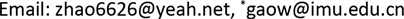Copyright © 2018 by authors and Hans Publishers Inc.1. 引言

2. 非结构网格下格式的构造

2.1. 非一致网格下的NVSF的正则化

$\stackrel{^}{\varphi }=\frac{\varphi -{\varphi }_{U}}{{\varphi }_{D}-{\varphi }_{U}},\text{\hspace{0.17em}}\stackrel{^}{x}=\frac{x-{x}_{U}}{{x}_{D}-{x}_{U}}$

2.2. 对流项有界性准则

M. S. Darwish指出由Gaskell和Lau  提出的对流有界性准则CBC是适用于非结构网格的。这个准则在正则变化的基础上指出，对于一个具有有界性的格式，满足以下条件：

$\left\{\begin{array}{l}{\stackrel{^}{\varphi }}_{C}<{\stackrel{^}{\varphi }}_{f}=f\left({\stackrel{^}{\varphi }}_{C}\right)<1,\text{\hspace{0.17em}}\text{\hspace{0.17em}}\text{\hspace{0.17em}}0<{\stackrel{^}{\varphi }}_{C}<1\hfill \\ {\stackrel{^}{\varphi }}_{f}={\stackrel{^}{\varphi }}_{C},\text{\hspace{0.17em}}\text{\hspace{0.17em}}\text{\hspace{0.17em}}\text{\hspace{0.17em}}\text{\hspace{0.17em}}\text{\hspace{0.17em}}\text{\hspace{0.17em}}\text{\hspace{0.17em}}\text{\hspace{0.17em}}\text{\hspace{0.17em}}\text{\hspace{0.17em}}\text{\hspace{0.17em}}\text{\hspace{0.17em}}\text{\hspace{0.17em}}\text{\hspace{0.17em}}\text{\hspace{0.17em}}\text{\hspace{0.17em}}\text{\hspace{0.17em}}\text{\hspace{0.17em}}\text{\hspace{0.17em}}\text{\hspace{0.17em}}{\stackrel{^}{\varphi }}_{C}\le 0\hfill \\ {\stackrel{^}{\varphi }}_{f}={\stackrel{^}{\varphi }}_{C},\text{\hspace{0.17em}}\text{\hspace{0.17em}}\text{\hspace{0.17em}}\text{\hspace{0.17em}}\text{\hspace{0.17em}}\text{\hspace{0.17em}}\text{\hspace{0.17em}}\text{\hspace{0.17em}}\text{\hspace{0.17em}}\text{\hspace{0.17em}}\text{\hspace{0.17em}}\text{\hspace{0.17em}}\text{\hspace{0.17em}}\text{\hspace{0.17em}}\text{\hspace{0.17em}}\text{\hspace{0.17em}}\text{\hspace{0.17em}}\text{\hspace{0.17em}}\text{\hspace{0.17em}}\text{\hspace{0.17em}}\text{\hspace{0.17em}}{\stackrel{^}{\varphi }}_{C}\ge 1\hfill \end{array}$ (1)Figure 1. Three neighboring mesh points and the mesh face on unstructured meshes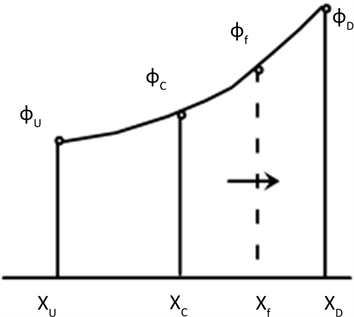Figure 2. The relationship between variables before the regularization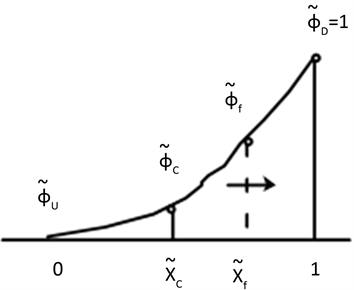Figure 3. The relationship between variables after the regularization

2.3. 新格式的构造

${\stackrel{^}{\phi }}_{f}=a{\stackrel{^}{\phi }}_{C}^{3}+b{\stackrel{^}{\phi }}_{C}^{2}+c{\stackrel{^}{\phi }}_{C}+d$ (2)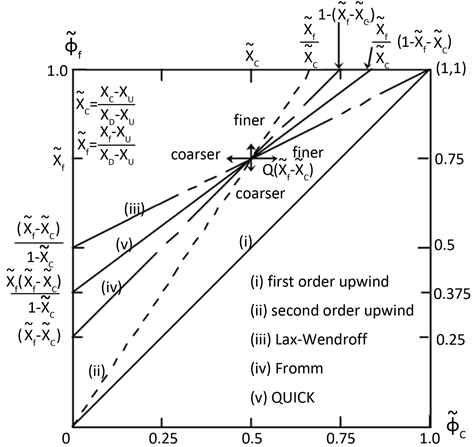Figure 4. Normalized Variable Diagram (NVD) for several linear schemes formulated using NVSF

$\left\{\begin{array}{l}{\stackrel{^}{\phi }}_{f}\left(0\right)=0\\ {\stackrel{^}{\phi }}_{f}\left({\stackrel{^}{x}}_{c}\right)={\stackrel{^}{x}}_{f}\\ {\stackrel{^}{\phi }}_{f}\left(1\right)=1\end{array}$ (3)

${{\stackrel{^}{\phi }}^{\prime }}_{f}\left({\stackrel{^}{x}}_{c}\right)=\frac{{\stackrel{^}{x}}_{f}\left({\stackrel{^}{x}}_{f}-1\right)}{{\stackrel{^}{x}}_{c}\left({\stackrel{^}{x}}_{c}-1\right)}$ (4)

$\left\{\begin{array}{l}a=\frac{{\left({\stackrel{^}{x}}_{c}-{\stackrel{^}{x}}_{f}\right)}^{2}}{{\stackrel{^}{x}}_{c}^{2}{\left({\stackrel{^}{x}}_{c}-1\right)}^{2}}\\ b=\frac{3{\stackrel{^}{x}}_{c}^{2}{\stackrel{^}{x}}_{f}+{\stackrel{^}{x}}_{c}{\stackrel{^}{x}}_{f}-2{\stackrel{^}{x}}_{c}^{3}-{\stackrel{^}{x}}_{c}{\stackrel{^}{x}}_{f}^{2}-{\stackrel{^}{x}}_{f}^{2}}{{\stackrel{^}{x}}_{c}^{2}{\left({\stackrel{^}{x}}_{c}-1\right)}^{2}}\\ c=\frac{{\stackrel{^}{x}}_{c}^{4}+{\stackrel{^}{x}}_{c}{\stackrel{^}{x}}_{f}^{2}+{\stackrel{^}{x}}_{c}{\stackrel{^}{x}}_{f}-3{\stackrel{^}{x}}_{c}^{2}{\stackrel{^}{x}}_{f}}{{\stackrel{^}{x}}_{c}^{2}{\left({\stackrel{^}{x}}_{c}-1\right)}^{2}}\\ d=0\end{array}$

$\begin{array}{c}{\stackrel{^}{\phi }}_{f}=\frac{{\left({\stackrel{^}{x}}_{c}-{\stackrel{^}{x}}_{f}\right)}^{2}}{{\stackrel{^}{x}}_{c}^{2}{\left({\stackrel{^}{x}}_{c}-1\right)}^{2}}{\stackrel{^}{\phi }}_{C}^{3}+\frac{3{\stackrel{^}{x}}_{c}^{2}{\stackrel{^}{x}}_{f}+{\stackrel{^}{x}}_{c}{\stackrel{^}{x}}_{f}-2{\stackrel{^}{x}}_{c}^{3}-{\stackrel{^}{x}}_{c}{\stackrel{^}{x}}_{f}^{2}-{\stackrel{^}{x}}_{f}^{2}}{{\stackrel{^}{x}}_{c}^{2}{\left({\stackrel{^}{x}}_{c}-1\right)}^{2}}{\stackrel{^}{\phi }}_{C}^{2}\\ \text{\hspace{0.17em}}\text{\hspace{0.17em}}+\frac{{\stackrel{^}{x}}_{c}^{4}+{\stackrel{^}{x}}_{c}{\stackrel{^}{x}}_{f}^{2}+{\stackrel{^}{x}}_{c}{\stackrel{^}{x}}_{f}-3{\stackrel{^}{x}}_{c}^{2}{\stackrel{^}{x}}_{f}}{{\stackrel{^}{x}}_{c}^{2}{\left({\stackrel{^}{x}}_{c}-1\right)}^{2}}{\stackrel{^}{\phi }}_{C}\end{array}$

${\stackrel{^}{\varphi }}_{f}={\stackrel{^}{\varphi }}_{C}^{3}-\frac{5}{2}{\stackrel{^}{\varphi }}_{C}^{2}+\frac{5}{2}{\stackrel{^}{\varphi }}_{C}$ (5)

3. 三角形剖分的变量关系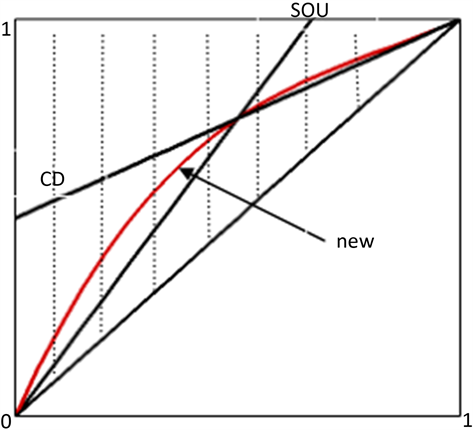Figure 5. New Format Curve and CBC Guidelines Area (area of dotted line)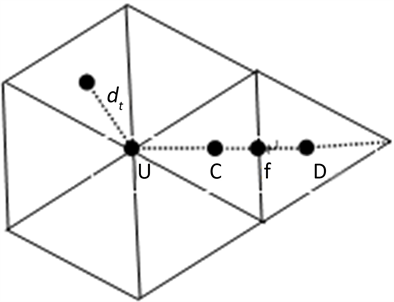Figure 6. The relationship between variables of the triangle mesh

${\phi }_{U}=\frac{\sum _{i=1}^{n}\frac{{\phi }_{i}}{{d}_{i}}}{\sum _{i=1}^{n}\frac{1}{{d}_{i}}}$

4. 时间的离散

$\left\{\begin{array}{l}{\varphi }^{\left(1\right)}={\varphi }^{n}+L\left({\varphi }^{\left(1\right)}\right)\hfill \\ {\varphi }^{\left(2\right)}=\frac{3}{4}{\varphi }^{n}+\frac{1}{4}\left[{\varphi }^{\left(1\right)}+L\left({\varphi }^{\left(1\right)}\right)\right]\hfill \\ {\varphi }^{n+1}=\frac{1}{3}{\varphi }^{n}+\frac{2}{3}\left[{\varphi }^{n}+L\left({\varphi }^{n}\right)\right]\hfill \end{array}$

5. 数值算例

5.1. 线性对流方程

$\frac{\partial \varphi }{\partial t}+\alpha \frac{\partial \varphi }{\partial x}=0$ , $x\in \left[a,b\right]$ , $t>0$ (6)

5.1.1. 情形1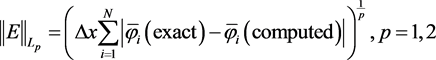$\text{order}=\frac{\mathrm{ln}{E}_{N}/{E}_{2N}}{\mathrm{ln}2}$

5.1.2. 情形2

$\varphi \left(x,0\right)=\left\{\begin{array}{c}1,\\ 0,\end{array}\begin{array}{c}x\le 0\\ x\ge 0\end{array}$

5.2. 二维线性对流方程Table 1. Errors and orders for several selected schemes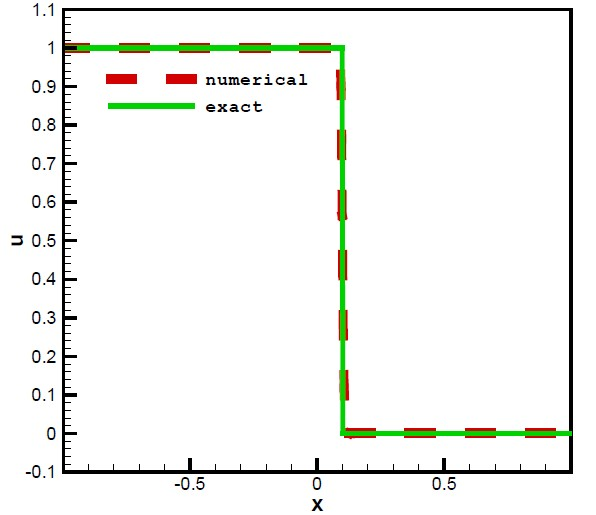Figure 7. Comparison of numerical and exact results for the new condition

$\frac{\partial \varphi }{\partial t}+\frac{\partial \left(a\varphi \right)}{\partial x}+\frac{\partial \left(b\varphi \right)}{\partial y}=0$

${\nu }_{t}\left(r\right)=\frac{1}{{\nu }_{\mathrm{max}}}\frac{\mathrm{tanh}\left(r\right)}{{\mathrm{cosh}}^{2}\left(r\right)}$

$a\left(x,y\right)=-\frac{y-{x}_{0}}{r}{\nu }_{t}\left(r\right)$ , $b\left(x,y\right)=\frac{x-{y}_{0}}{r}{\nu }_{t}\left(r\right)$

$\varphi \left(x,y,t\right)=\mathrm{tanh}\left[\frac{y-{x}_{0}}{\delta }\mathrm{cos}\left(\omega t\right)-\frac{x-{y}_{0}}{\delta }\mathrm{sin}\left(\omega t\right)\right]$

6. 结论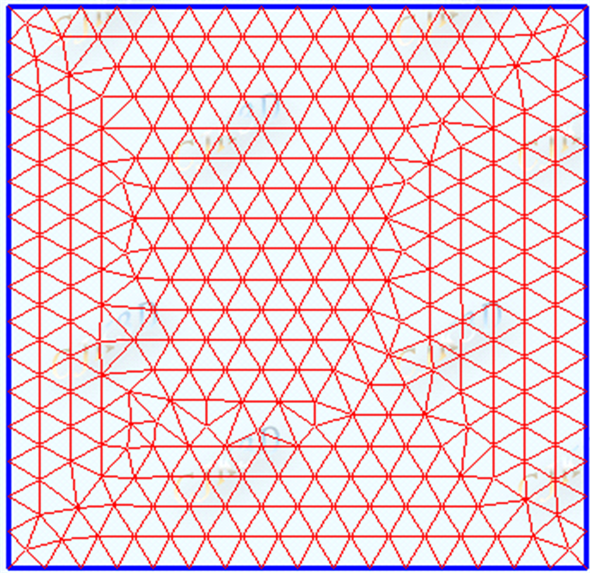Figure 8. Triangulation diagram of EasyMesh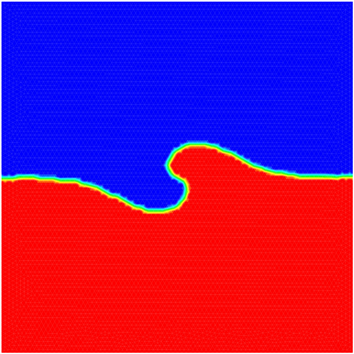(a)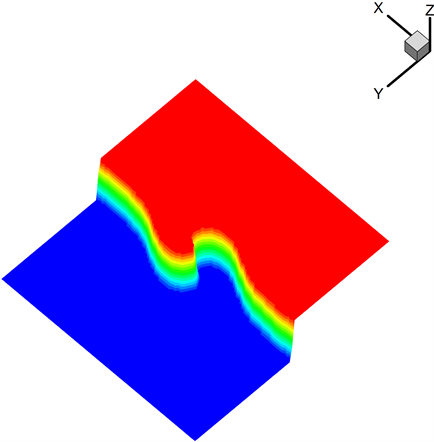(b)

Figure 9. Exact and numerical solutions of Doswell

A Non-Oscillatory Scheme for Convection Diffusion Equations on Triangular Meshes[J]. 流体动力学, 2018, 06(02): 23-32. https://doi.org/10.12677/IJFD.2018.62004

1. 1. Gottlieb, S. and Shu, C.-W. (1998) Total Variational Diminishing Runge-Kutta Schemes. Mathematics of Computation, 67, 73-85. https://doi.org/10.1090/S0025-5718-98-00913-2

2. 2. Van Leer, B. (1977) Towards the Ultimate Conservative Difference Scheme. V. A Second-Order Sequel to Godunov’s Method. Journal of Computational Physics, 23, 101-136.

3. 3. Lenard, B.P. (1988) Simple High-Accuracy Resolution Program for Convective Modeling of Discontinuities. International Journal for Numerical Methods in Fluids, 8, 1291-1318. https://doi.org/10.1002/fld.1650081013

4. 4. Doswell, C.A. (1998) A Kinematic Analysis of Frontogenesis Associated with a Non-Divergent Vortex. Journal of the Atmospheric Sciences, 41, 1242-1248. 2.0.CO;2>https://doi.org/10.1175/1520-0469(1984)041<1242:AKAOFA>2.0.CO;2

5. 5. Gaskell, P.H. and Lau, A.K.C. (1988) Curvature-Compensated Convective Transport: SMART, A New Boundedness-Preserving Transport Algorithm. Interna-tional Journal for Numerical Methods in Fluids, 8, 617-641. https://doi.org/10.1002/fld.1650080602

6. 6. Van Leer, B. (1974) Towards the Ultimate Conservative Difference Scheme: II. Monotonicity and Conservation Combined in a Second-Order Scheme. Journal of Computation Physics, 14, 361-370. https://doi.org/10.1016/0021-9991(74)90019-9

7. 7. Wei, J.J., Yu, B., Tao, W.Q., Kawaguchi, Y. and Wang, H.S. (2003) A New High-Order-Accurate and Bounded Scheme for Incompressible Flow. Numerical Heat Transfer, Part B: Fundamentals, 43, 19-41. https://doi.org/10.1080/713836153

8. 8. Alves, M.A., Oliveire, P.J. and Pinho, F.T. (2003) A Convergent and Universally Bounded Interpolation Scheme for the Treatment of Advection. International Journal for Numerical Methods in Fluids, 41, 47-75. https://doi.org/10.1002/fld.428

9. 9. Chakravarthy, S.R. and Osher, S. (1983) High Resolution Applications of the OSHER Upwind Scheme for the Euler Equations. AIAA Paper 83-1943. https://doi.org/10.2514/6.1983-1943

10. 10. Darwish, M.S. and Moukallod, F.H. (1994) Normalized Variable and Space Formulation Methodology for High-Resolution Schemes. Numerical Heat Transfer, Part B, 26, 79-96. https://doi.org/10.1080/10407799408914918

11. 11. Spalding, D.B. (1972) A Novel Finite Dif-ference Formulation for Differential Expressions Involving Both First and Second Derivatives. International Journal for Nu-merical Methods in Engineering, 4, 551-559. https://doi.org/10.1002/nme.1620040409

12. 12. Leonard, B.P. (1987) SHARP Simulation of Discontinuities in Highly Con-vective Steady Flow. NASA Technical Memorandum 100240, ICOMP-87-9.